# SBI PO Prelims Exam 2015: Concept and Sample Questions: Data Sufficiency

The banking team of jagranjosh.com has come up with concept and sample questions for Data Sufficiency. The concept provided by us will help you to understand the topic.

May 16, 2015 17:45 IST

The core of data sufficiency question is similar to Maths or Quantitative Comparison (QC) questions, but the scope and relative frequencies of the problem type vary. Importantly, knowledge of mathematics is of little or no use in preparing for these questions.

Data Sufficiency problem can be considered to have 3 parts

1. Original Information
2. Question stem
3. Statements

Let us discuss each part one by one:

Original information

Original information is the information given at the beginning of the question including the diagram (if any), which is to be used in considering both of the statements. In one quarters to one half of the problems, there will be no given information separate from the question.

When a diagram is a part of the given information, it will conform to the rest of the given information but might not conform to one or both of the statements.

Question Stem

The questions that are asked in DS problems can be divided into two types according to the type of answer they require. The first type asks for a specific number as an answer.

Secondly type of questions requires ‘yes’ or ‘no’ as an answer. Usually one third of the questions will ask yes/no questions; the remainder will ask for specific number.

Statements

The two statements can contain any sort of information that is appropriate to the problem and could even have diagram associated with them, though that is rare. Each statement will give a particular fact or describe a relationship, even two facts or relationships.

Measures of Central Tendency

The most common measures of central tendency are:

1. Arithmetic Mean (AM)
2. Weighted Arithmetic Mean
3. Median
4. Mode
5. Geometric Mean (GM)
6. Harmonic Mean (HM)

Mean

• Ungrouped Data

If x1, x2, x3, ……………………………………….xare the n numbers, then the mean if numbers will be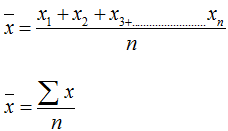• Grouped Data

In the frequencies of variables x1, x2, x3……………………………..xn are f1, f2, f3……………………………………fn respectively the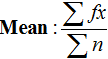Median

If the n values in a raw data are arranged in ascending or descending order. Then, the middle value is called the median.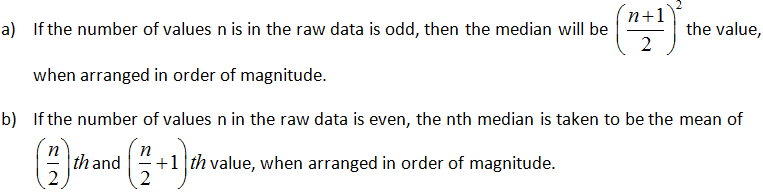Mode

The value which occurs most often in a data is the mode. In the other words, the data value having the highest frequency is called mode.

(i) Mode of ungrouped data x1,x2,x3,…,xn are the given data values and a value xk is repeated maximum number of times, that is, xk has the highest frequency, then the mode is xk.

(ii) Mode of grouped data Locate the class having the highest and the lowest frequency in the frequency table. The class having the maximum frequency table. The class having the maximum frequency is called the model class and the mode of such distribution is given by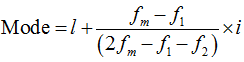Where, l = lower limit of the model class

fm = frequency of the model class (maximum frequency)

f1  = frequency just before ht model class

f2 = frequency just after the model class

i = width of the class interval

An important relation: An approximate relation between the arithmetic mean, medium, and mode for a given data distribution is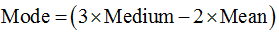Geometric Mean (GM)

(i) GM for ungrouped data Let x1,x2,….xn be the n given data values. Then

GM = (x1,x2,x3…xn)1/n

(ii) GM of grouped data Let data values x1,x2,….xn have respective frequencies f1,f2,f3….fn; now if G is the geometric mean, then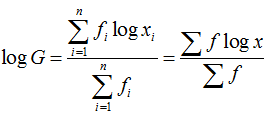Note: Geometric mean is used to obtain the rate of population growth, rate of interest, and in the formation of index numbers.

Harmonic Mean (HM)

(i) HM of ungrouped data Let x1,x2,x3,…,xn be the given data values, Then the harmonic mean H is given by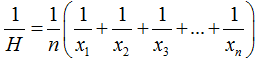(ii) HM of grouped data let x1,x2,x3,…xn have respective frequencies on f1,f2,f3….fn then the Harmonic mean H is given by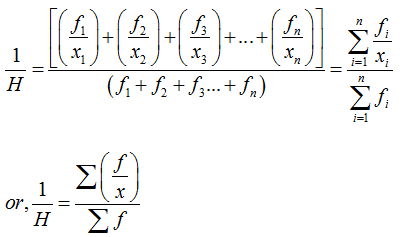Note: (a) If A is the arithmetic mean, G the geometric mean, and H the harmonic mean of a data distribution, then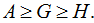(b) Harmonic mean proves useful in cases such as finding the average speed when the speed for different parts of journey are given as distance per unit time.

Formulas for Statistics

1. Crude Birth Rate: It is defined as the number of births per 1,000 of the population.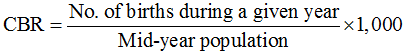2. Crude Death Rate: It is defined as the number of deaths per 1,000 of the population.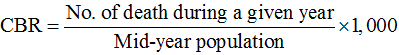3. Specific Death Rate: It is defined as the number of deaths per 1,000 of the population in a specified class in a given year.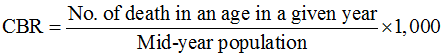4. Infant Mortality Rate: It is an important specific death rate. It is the number of infants under one year of age dying in a year per 1,000 live births in the same year.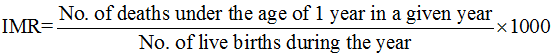5. Standarized Death Rate: It is given by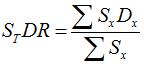Where, Sx = Standardized population for group x

and Dx = Specific death rate for group x

6. Price Index Number: It is given by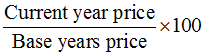7. Cost of Living Index: It is given by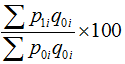Where, p0i = Price of a commodity in the base year

p1i = Price of commodity in the current year

q0i = Quantity of the commodity consumed in the base year.

Median

If the n values in a raw data are arranged in ascending or descending order. Then, the middle value is called the median.